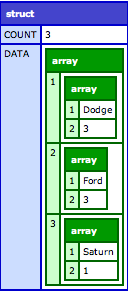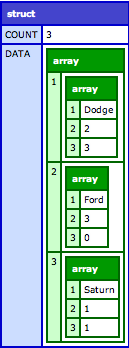In my last post, I showed how you can pretty easily integrate ColdBox’s awesome CriteriaBuilder with subqueries. As I was using this approach, I came across another (unrelated) challenge that I wanted a solution for.

## Simple Grouping/Summing

In one of my queries, I wanted to do some simple grouping/summing. With CriteriaBuilder, you can do this very easily by adding a projection or two.

For example, let’s imagine that we have the same entities as before:

• Car
• Driver
• Insurance

This time, we want to get a sum of the total number of cars, grouped by “make”. Easy:```public struct function getCarsByMake() {
var c  = newCriteria();
c.withProjections( count="CarID", groupProperty="Make" );
var results = {
data = c.list()
};
results.count = arrayLen( results.data );
return results;
}```

And the SQL:

```select this_.Make as y0_, count(this_.CarID) as y1_
from   Car this_
group by this_.Make```

Pretty simple. By the application of the “count” and “groupProperty” projections, we get back a nice array of the grouped data. Note, of course, that because of the application of projections, our result set is no longer in the domain of discreet entities. Rather, since we asked for aggregate data, that’s what we get! Of course, this means that results.count has to be calculated differently. Instead of running CriteriaBuilder’s count() method (which will ignore the projections), I just count the total size of the array of aggregate data that is returned. Clear enough? Onward!

## The Challenge

The example above is pretty stripped down, out-of-the-box goodness. With absolutely no customizations required, I get back some really nice transformations of the data.

Of course, it’s never quite that easy, right? Imagine that I wanted to do something a bit more complicated, such as:

• Return total count of cars LESS THAN 2012 model year, grouped by Make
• Return total count of cars GREATER THAN 2006 model year, grouped by Make

Obviously, we could run several queries with the criteria for each case. But what if we wanted this all back in one result?

In SQL, you can easily do something like so:

```select Make,
(select count(*) from Car c where Year <; 2012 and c.Make = Car.Make) as TotalPre2012,
(select count(*) from Car c where Year >; 2006 and c.Make = Car.Make) as TotalPost2006
from  Car
group by Make```

But what about CriteriaBuilder? In ColdBox, the relevant projection (e.g., count) only takes a propertyName–there’s no way to more granularly restrict the value, outside of adding a criterion (which would, back to the original point, require multiple queries for the desired result).

Fortunately, the Hibernate criteria query API has two handy projections–sqlProjection and sqlGroupedProjection–that make this super-easy to accomplish.

## SqlProjection and SqlGroupedProjection

Both projections have a similar structure–in fact, the only real difference is that sqlGroupedProjection, true to its name, allows for you to specify which property(ies) you’d like to group on. Here’s a breakdown of what they require:

• sql: arbitrary SQL to be executed (can be subquery, etc.)
• groupBy: if using sqlGroupedProjection, this is the field(s) which will be added to the executed SQL for grouping
• columnAliases: array of aliases being used in the arbitrary SQL. So if you have something like count( * ) as Total, you should use “Total” as one of the aliases
• types: array of types of the columns that are being projected

To make this a bit easier to do, I simply extended ColdBox’s CriteriaBuilder withProjections() method a bit to incorporate these two. Here’s what sqlProjection looks like:

```// sqlProjection
if( structKeyExists( arguments, "sqlProjection" ) ) {
var sqlargs = arguments.sqlProjection;
// allow for either an array of sqlProjections, or a stand-alone config for one
if( !isArray( sqlargs) ) {
sqlargs = [ sqlargs ];
}
// loop over sqlProjections
for( var projection in sqlargs ) {
var propertyTypes = [];
// retrieve correct type for each specified property so list() doesn't bork
for( var prop in listToArray( projection.property ) ) {
arrayAppend( propertyTypes, metaData.getPropertyType( prop ) );
}
// add sqlProjection to the overall projectionList
projection.sql,
listToArray( projection.alias ),
propertyTypes
), projection.alias );
}
}```

Now that I’ve done that, I can simply use this new projection to achieve my original objective:

```public struct function getProjectionGroupedMakeBreakdown() {
var c  = newCriteria();
var sql = [
{
sql = "(select count(*) from Car where Year <; 2012 and Make={alias}.Make) as TotalMakePre2012",
alias = "TotalMakePre2012",
property="Year"
},
{
sql = "(select count(*) from Car where Year >; 2006 and Make={alias}.Make) as TotalMakePost2006",
alias = "TotalMakePost2006",
property="Year"
}
];
c.withProjections( groupProperty="Make", sqlProjection=sql );
var results = {
data = c.list()
};
results.count = arrayLen( results.data );
return results;
}```

NOTE: The use of {alias} above is pretty simple. It’s simply a reference to the root entity. Since our root entity is currently “Car”, {alias} is transformed in the generated SQL to “this_”.

This gives me:And the SQL:

```select this_.Make as y0_,
(select count(*)  from Car  where Year <; 2012 and Make=this_.Make) as TotalMakePre2012,
(select count(*) from Car where Year >; 2006 and Make=this_.Make) as TotalMakePost2006
from Car this_
group by this_.Make```

Of course, I could have also done this to combine the projections:

```var sql = {
sql = "(select count(*) from Car where Year <; 2012 and Make={alias}.Make) as TotalMakePre2012,
(select count(*) from Car where Year >; 2006 and Make={alias}.Make) as TotalMakePost2006",
alias = "TotalMakePre2012,TotalMakePost2006",
property="Year,Year"
};```

Either way, I get back a nice, aggregated array of data that gives me:

• The make of the vehicle (grouped)
• The number of vehicles for the particular make that were made prior to 2012
• The number of vehicles for the particular make that were made after 2006

## Conclusion

Using sqlProjection and sqlGroupProjection is pretty cool, but I’m still a bit unsatisfied. Even though this approach allows me to get at what I need, it’s still a bit “hard-coded” for my tastes. To make this dynamic, I’m going to have to do some kind of string building (yuk). I’m currently looking into other ways to accomplish this, but at the moment I’m at an empasse. If you know of anything, let me know!!